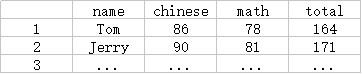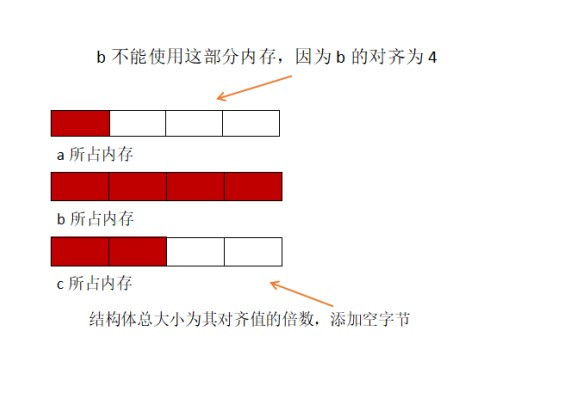# struct（结构体）

在实际问题中，一组数据往往具有不同的数据类型。例如， 在学生登记表中，姓名应为字符型（char[])；学号可为整型(int)或字符型(char[])；年龄应为整型(char)；性别应为字符型(char)；成绩可为整型(int)或实型(double)。 显然不能用一个数组来存放这一组数据。 因为数组中各元素的类型和长度都必须一致，以便于编译系统处理。为了解决这个问题，C语言中给出了另一种构造数据类型——“结构”。

“结构”是一种构造类型，它是由若干“成员”组成的。 每一个成员可以是一个基本数据类型或者又是一个构造类型。 结构即是一种“构造”而成的数据类型， 那么在说明和使用之前必须先定义它，也就是构造它。如同在说明和调用函数之前要先定义一样。

#### 1.定义结构体及结构体变量

struct 结构体类型名//struct是关键字

{

}结构变量量表；//可以同时定义多个结构体变量用“,”隔开，另外结构变量表后面的“;”不能漏掉。例如：

struct student//定义一个名叫student的struct类型
{
string name;//定义名字
int chinese;//定义语文成绩
int math;//定义数学成绩
int total;//定义总成绩
}a;//同时定义了a的数组变量
//也可以下面这样：
struct student
{
string name;
int chinese;
int math;
int total;
};
student a;//这里同样可以定义多个结构体变量

#### 2.结构体变量的特点

(1).结构体变量可以整体操作。例如；
swap(a[i],a[i+1]);
(2).结构体变量的成员的访问也很方便、清晰。例如：
cin>>a[i].name>>a[i].chinese>>a[i].math;
(3).结构体变量的初始化和数组的初始化类似。例如：
student op={"gaoxiang",80,90,170};

#### 3.成员调用

cin>>a[i].name;                            //一般形式不能写cin>>a[i];
a[i].tatal=a[i].chinese+a[i].math;  // 就像整形变量一样使用

student.abc.menbb

结构体变量abc有成员menbb.

结构体变量名.成员函数

#### 5.sort排序的使用

bool cmp(结构体类型名 x,结构体类型名 y)
{
return x.结构体变量名>y.结构体变量名;
}

bool cmp(struct x,struct y)
{
return x.total>y.total;
}

#### 5.struct的内存储存方式

结构体 struct 是多个相互关联数据的集合，这些数据以整体形式存储在内存。

struct A
{
char  a;      //char占1个字节
int   b;      //int 占4个字节
short c;      //short占2个字节
};

1.数据类型对齐值:即为sizeof的值。
2.结构体对齐值:结构体中对齐值最大类型的对齐值。
3.结构体总大小为 其对齐值得增数倍，需要时编译器会自动添加空字节。
4.结构体元素按顺序分配内存
按照上述规则：a在第0个字节开始，占一个字节;b则在第4个字节开始,占4个字节;c在第8个字节开始，占2个字节。为使结构体总大小为其对齐值得倍数，添加两个空字节，结构体总大小为12.

#### 6.题目解释

n行：每行格式有：姓名 语文 数学 英语 总分

5
gaoxing 78 96 80
wangxi 70 99  69
liujia 90 87 77
zhangjin 78 91 67
tom 89 87 78

gaoxing 78 96 80 254
liujia 90 87 77 254
tom 89 87 78 254
wangxi 70 99 69 238
zhangjin 78 91 67 236

#include<bits/stdc++.h>//万能头文件
using namespace std;
struct A
{
string name;//姓名
int chinese;//语文
int math;//数学
int english;//英语
int total;//总成绩
int num;//序号
}s;//结构体
bool cmp(A x,A y)//判断比较
{
return x.total==y.total? (x.math==y.math? x.num<y.num:x.math>y.math):x.total>y.total;
//判断 按总分从高到低排序，总分数相同按数学成绩的高低排序，两者相同按输入顺序排序。
}
int main()
{
int n;
cin>>n;
for(int i=1;i<=n;++i)//输入
{
cin>>s[i].name>>s[i].chinese>>s[i].math>>s[i].english;
s[i].total=s[i].chinese+s[i].math+s[i].english;
s[i].num=i;
}
sort(s+1,s+n+1,cmp);
for(int i=1;i<=n;++i)//输出
{
cout<<s[i].name<<" ";
cout<<s[i].chinese<<" ";
cout<<s[i].math<<" ";
cout<<s[i].english<<" ";
cout<<s[i].total<<endl;
}
return 0;
}


09-051万+08-162万+
05-212540
07-091万+
12-219815
04-296万+
09-116262
06-242万+
04-224920
07-123万+